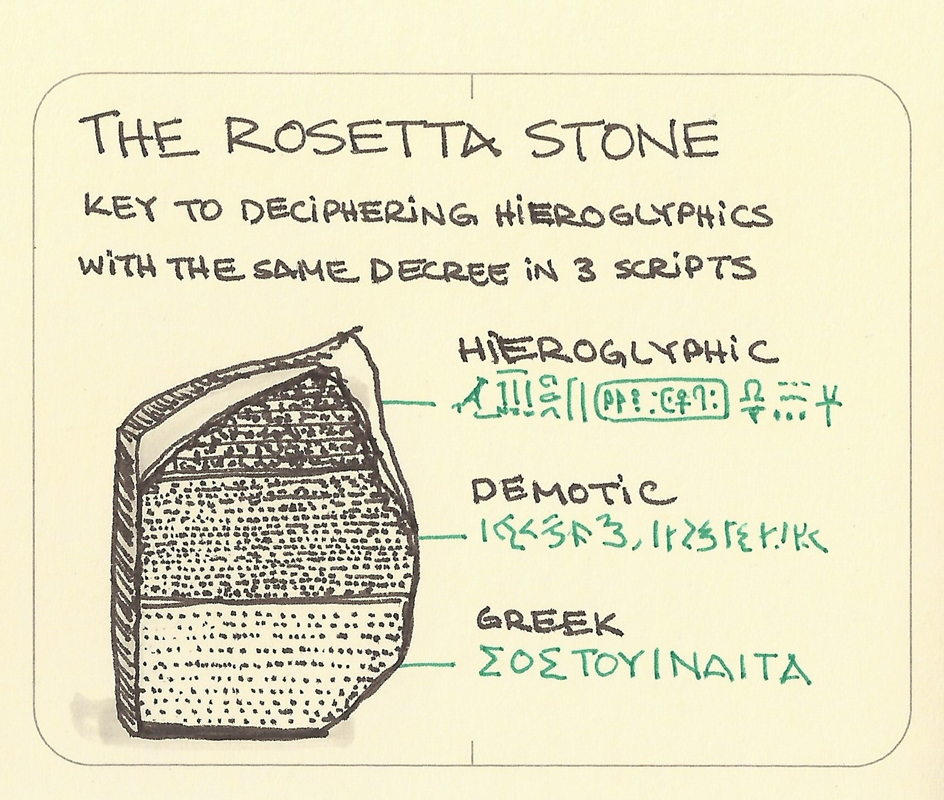# Porting equations from Excel to SolidWorks

I develop a lot of equations for use in my designs. Math is behind most of what I make. Generally, I’m using MS Excel while going through the math and drawings to arrive at the system that I want to use. That gets added to master spreadsheets along with all my other calculations that I’ve developed over time. Then I port those to SolidWorks models for the projects that I do.

In figuring out the settings calculation spreadsheet for my recent frame fixture, I was forced to use a few functions in Excel to help me arrive at the values I needed. These were generally rounding from the exact values.

=CEILING(C83,C80)

=FLOOR((C83,C80)

=IF(C88>0, ROUNDDOWN(C83,0),ROUNDUP(C83,0))

These are just a couple of examples and should have no real meaning to anyone. The important thing to see here is the several functions that I’m using. There are plenty of others but these are ones that came up.

The problem is, we can’t use those functions in SolidWorks. The functions that we have available are very limited in the native equation editor. There really should be some more options but we have a precious few.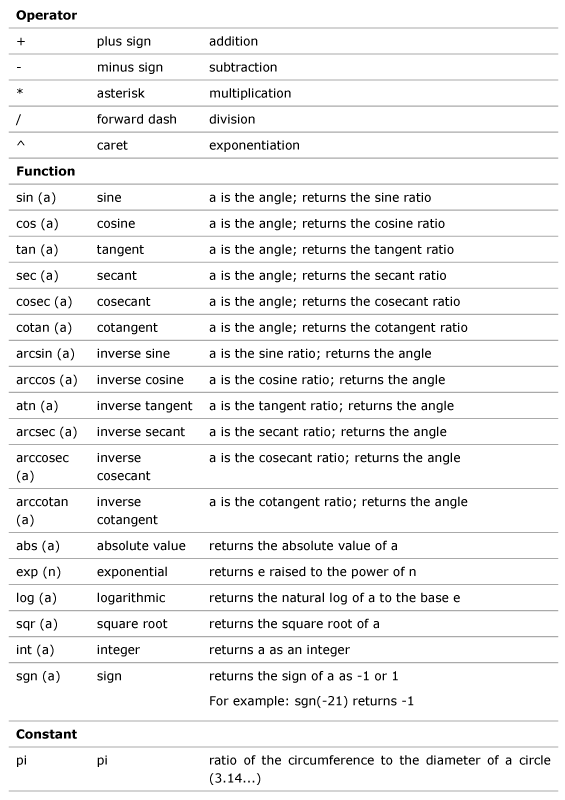For some crazy reason, two functions have been left from the published list; IFF() (or IF) function and ROUND(). That’s a dam shame as IFF() is an incredibly powerful tool. We can use the IFF() function to help automate decisions within the equation editor. We can also use it to construct functions to help make up for lacking many of the handy functions that we use in Excel.

Here’s the form that most folks know:

=IFF (evaluation, true return, false return)

This is clear and obvious to anyone familiar with spreadsheets or computer programming; If the statement is true, return THIS, otherwise, return THIS. This is probably one of the most powerful things that we can have at our disposal, we just need to use it well.

In both programs, the IF/IFF function can be very deeply nested to split the path in several directions

RearRotorFromCenter = IF(RearHubSpacing=148,55.25,IF(RearHubSpacing=142,52.25,IF(RearHubSpacing=110,44.5,IF(RearHubSpacing=100,39.5, “FAIL”))))

“Rear Rotor from Center” = IIF ( “Rear Axle Spacing” = 148 , 55.25 , IIF ( “Rear Axle Spacing” = 142 , 52.25 , IIF ( “Rear Axle Spacing” = 110 , 44.5 , 39.5 ) ) )

= ROUND ( Value )

This rounds as typical. Just like you learned in school.

Now we get to the serious problem that hid from me for a while, the INT() function. While it would seem like a no brainer to use this just as we do in Excel, we can’t. For some utterly spellbinding reason the folks at Dassault Systèmes have made their INT() work very differently from MS Excel INT().

In Excel, INT() rounds a evaluation value to the next lowest integer. If you have a value of -5.123, the function will round it to -6. Simple. This is what we are used to. I’m not saying that this is correct or not, just that in the last 40 years and billions of Excel users have come to learn that this is what happens.

In SolidWorks, INT() rounds the evaluation value to the next lowest integer if the evaluation value is positive. If the evaluation value is negative, it will round to the next highest integer. That means that a value of -5.123 will be rounded to -5.

This is fucking insane. This is what, in the shop, I call a booby-trap. Anyone working with computer systems would expect this to work as it does in Excel but it doesn’t and not in a way that you would catch if you weren’t paying attention. Fuck, I didn’t even figure this out until I was preparing this post. That’s unreal and actually makes me angry.

Now, we need to understand the difference between a computer programmer and a user. A computer programmer will see INT() just as SolidWorks does. I show this below. A MS Office user or VBA user will see it as Excel does. I certainly don’t believe that the computer programmers should win this battle as this is an application and we are users.

What we need to be very clear on is that INT() in SolidWorks is the equivalent of ROUNDDOWN() in Excel. This causes quite a mess and all kinds of possibilities for error. Regardless, it is what it is and we just have to be clear of that.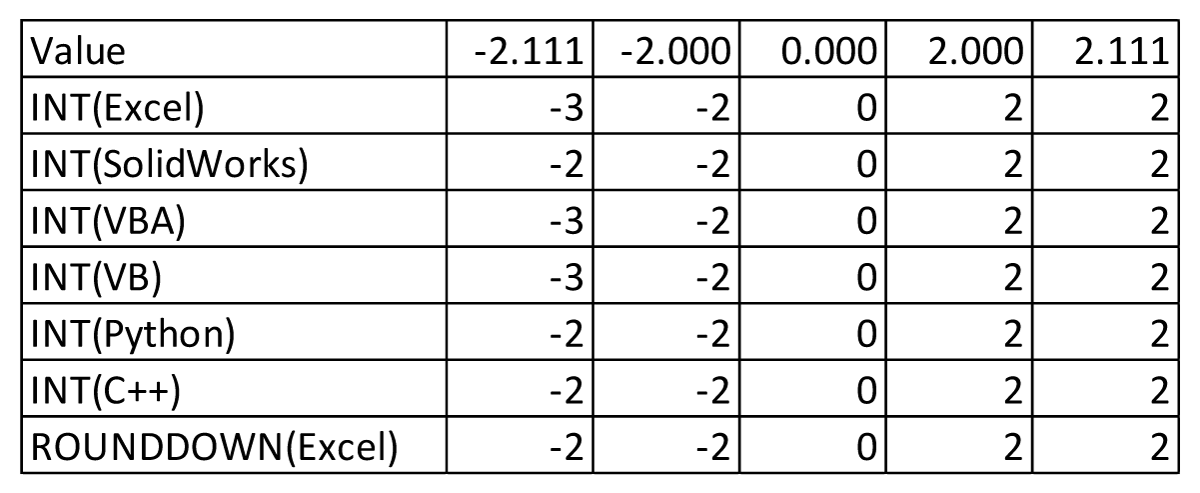There is another real problem in SolidWorks equations that folks should be very aware of. When working with IFF() and inequalities, you may not use “<=”, “>=”, “=<“, or “=>”. It may seem like it works but if you examine the values that are produced, they may be wrong. I, again, found this while preparing this post. I had a “>=” in a formula and the INT() in part of the IFF() options worked incorrectly. I had no idea but only found it as my Excel values weren’t matching with SolidWorks.

You can test this yourself, try: “= IIF ( “Value” > = 0 , INT ( “Value” ) , INT ( “Value” ) )” with “Value” = -2.111. You’ll get a value of -3…but INT(-2.111) in SolidWorks should be -2. Replace the “>=” with “>” and you get a value of -2. That’s fucking insane. Holy crap!

So, let’s look at some SolidWorks equations in preferred formats to understand what we need to do in Excel:

Rounding up:

“Value2” = IIF ( “Value” – INT ( “Value” ) > 0 , INT ( “Value” ) + 1  , INT ( “Value” ) )

Rounding down:

“Value2” = IIF ( “Value” – INT ( “Value” ) < 0 , INT ( “Value” ) – 1 , INT ( “Value” ) )

Rounding toward zero:

“Value2” = INT (“Value”)

This is all wonderful…until we realize that we didn’t want integers…we wanted hundredths. Simple enough, just scale the values. For example:

Rounding up, hundredths:

“Value2” = IIF ( “Value” – INT ( “Value” ) > 0 , ( INT ( “Value” * 100 ) + 1 ) / 100 , INT ( “Value” * 100 ) / 100 )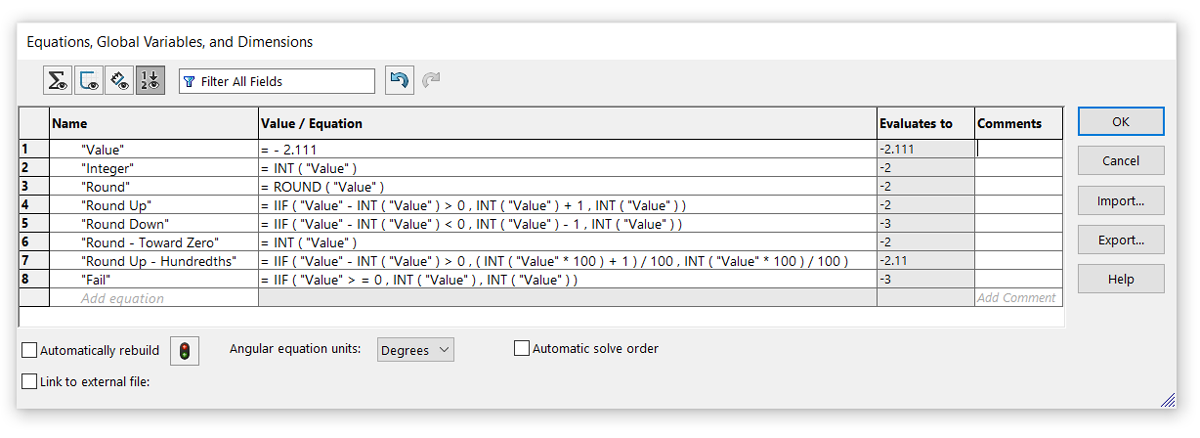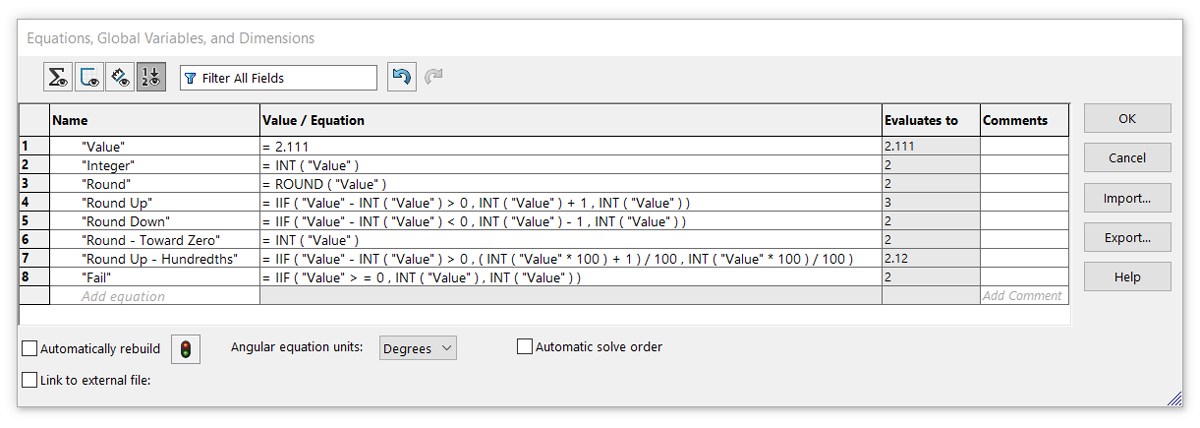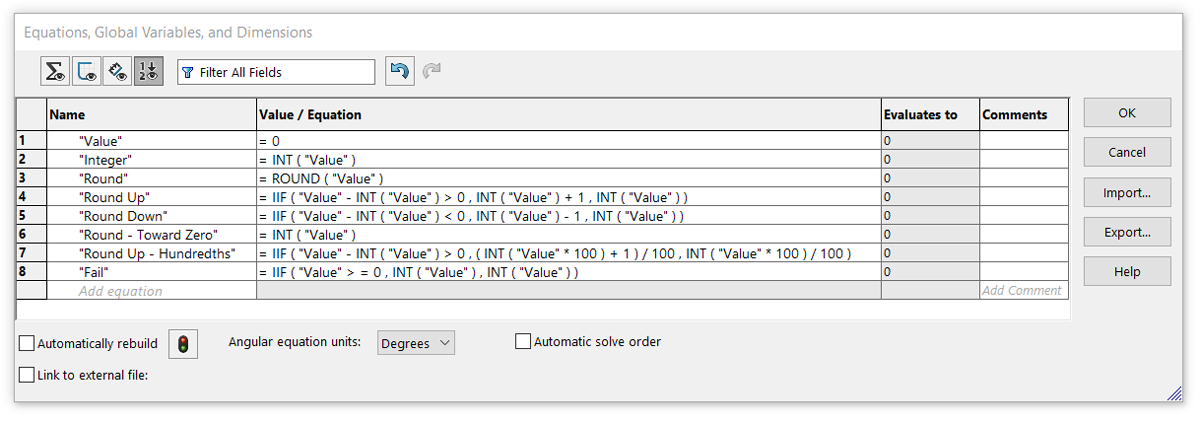In Excel, the formula are the same but the function naming and parameters need changing. This makes for a bit of a mess in conversion but it is what we have to do.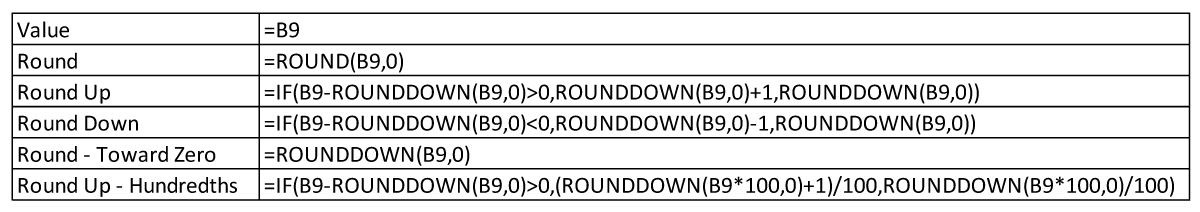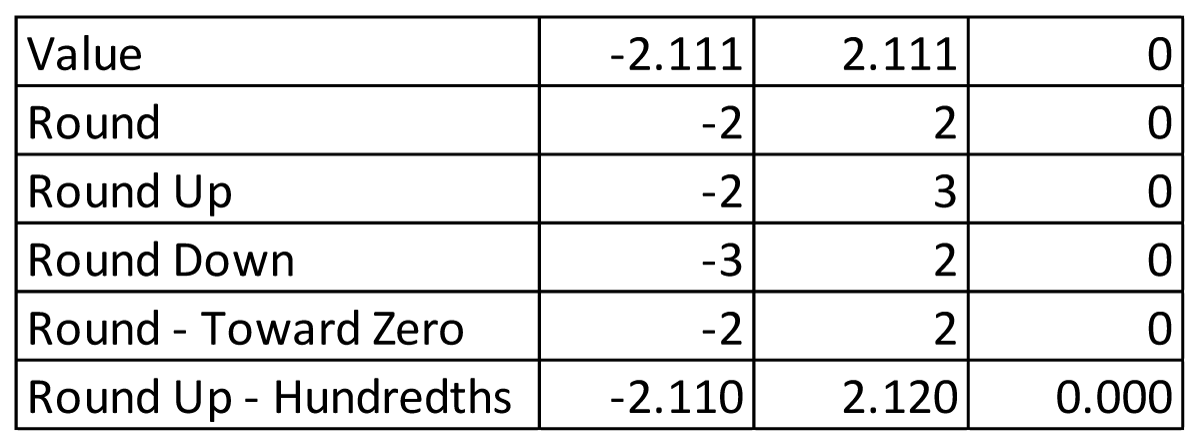I know that this isn’t what folks want to learn. I would prefer many of the tools we’ve grown accustomed to in any spreadsheet to be available in SolidWorks. I think it really is one of the most un-developed parts of the software and that’s a real shame.